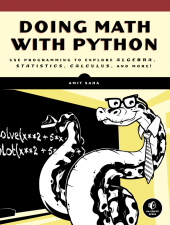Doing Math with Python is written by Amit Saha and published by No Starch Press. It has been translated to French, Simplified Chinese, Korean and Japanese.

The English version of the book is now available in print and electronic formats (including Amazon Kindle). Please refer to the Buy page. Some readers have taken the time out to post a review of the book on Amazon and elsewhere. Please refer to the Reviews page.There are seven chapters and 2 Appendices in the book. A detailed table of contents is available from the publisher's website.

## Chapter 1: Numbers

This chapter starts with the absolute basics of handling numbers in Python. It discusses integers, floating point numbers, fractions, complex numbers and performing operations with them.

## Chapter 2: Visualizing Data with Graphs

This chapter introduces Matplotlib and shows how to create graphs representing collections of numbers.

## Chapter 3: Describing Data with Statistics

As the title suggests, this chapter is all about the statistical measures one would first learn in high school – mean, median, mode, frequency table, range, variance, standard deviation and linear correlation are discussed.

## Chapter 4: Algebra and Symbolic Math with SymPy

The first three chapters are all about number crunching. The fourth chapter introduces the reader to the basics of manipulating symbolic expressions using SymPy. Factorizing algebraic expressions, solving equations, plotting from symbolic expressions are some of the topics discussed in this chapter.

## Chapter 5: Sets and Probability

This chapter starts off with how to create a set and demonstrating the common set operations. Utility of the different set operations are demonstrated via simple applications. For example, Cartesian product is used to write a program to simulate an experiment to calculate the time period of a simple pendulum of different lengths and at places with varying gravity. Union and intersection operations are applied to finding the probability of events.

The chapter then moves onto discussing how to generate uniform and non uniform random numbers, and using them to simulate scenarios such as a die roll and a fictional ATM which dispenses dollar bills of different denominations with varying probability.

## Chapter 6: Drawing shapes and Fractals

This chapter is logically divided into two parts. The first part introduces the reader to matplotlib patches which allows drawing geometric shapes (circles and polygons), followed by matplotlib’s animation API which allows drawing animated figures. The trajectory of a projectile motion discussed elsewhere in various contexts is animated combining both these things.

The second part of the chapter introduces the concept of geometric transformation. Combining that with the knowledge of generating random numbers learned earlier in Chapter 5, the reader will learn how to draw fractals such as the Barnsley Fern, Sierpinski triangle and Mandelbrot set.

## Chapter 7: Solving Calculus Problems

This chapter discusses solving differentiation and integration tasks using programs. As applications, the gradient ascent (and descent) methods and continuous probability are discussed.

## Appendix A

This appendix covers the installation of software required to run the programs in the book on Microsoft Windows, Linux and Mac OS X.

## Appendix B

This appendix discusses some Python topics which the reader may not be familiar with, but are needed to follow some parts of the book. It also includes topics which provide additional information beyond that required for the programs in the book.

### Stay in touch

You can stay connected with the book, its readers and me via the following channels:

You can contact me directly via:

Page last updated: August 29, 2015